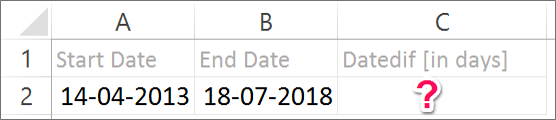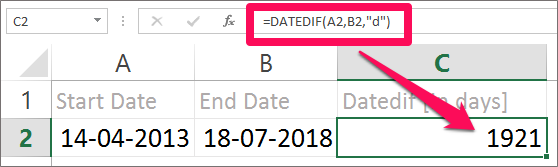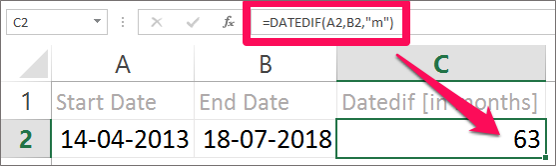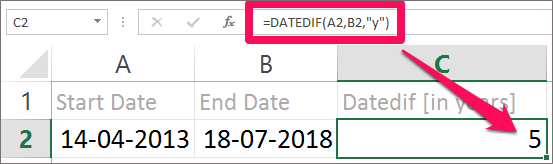Need Help? Chat with us

DATEDIF Function in Excel

DATEDIF Function in Excel

DATEDIF function as the name itself explains the difference between the dates. This function is used to calculate the difference in dates by days, months or years according to the interval specified in the syntax.

The DATEDIF function is a worksheet function and it returns a numeric value in return.

The DATEDIF function is an in-built function in Excel. However, this function is one of the rare function that is not documented while we enter it in the worksheet.

It was only visible in the Excel 2003 version. It is available in all the version and is also used for calculation it is just not documented.

Note: Excel won’t let you fill the arguments as it did for other function, but it will work when correctly configured.

In other words, DATEDIF function in Excel calculates the difference between the start date and the end date in days, months and years. It is said that DATEDIF function is a hidden function in Excel.

DATEDIF(Start_Date, End_Date, Unit)

Where,

Start_Date is the starting date of the period you want to calculate.

End_Date is the end date of the period you want to calculate.

Unit is the interval you want to calculate the difference. The unit and their respective explanation are explained below:

 Unit Explanation Y The number of years completed M The number of months completed D The number of days completed MD The difference in days (Months and Years are ignored) YM The difference in months (Years and Days are ignored) YD The difference in days (Years and Months are ignored)

It is compulsory to enter all the three arguments that is required to calculate the DATEDIF function in Excel.

NOTE: The Start Date should not be greater than the End Date.

Below are the detailed Example of each unit which will clear your image regarding the DATEDIF in Excel.

DATEDIF Function Example

1. Calculating the number of days completed between the two dates.

We need to find the number of days between the dates specified. Follow the steps to calculate the number of days between the start date and the end date.

Step 1: In a data set, enter the start date and the end date it different columns or rows. (Here we have entered a Start date in cell A2 and End date in cell B2.)Step 2: Start the formula with and “=” equal to sign. (We are entering the formula in cell C2.)

Step 3. Press Enter. Results will be displayed.(Here the Answer will be 1921 days.)

2. Calculating the number of months completed between the two dates.

While Calculating the number of months completed between the two dates, all the other arguments remain the same as you did above while calculating the number of days between two dates. Expect in the Unit argument instead of writing “d” you need to replace it will “m”.(Here the Answer will be 63 Months)

Note: DATEDIF will show a #NUM error if start date is greater than end date.

3. Calculating the number of years between two dates.

Fill in the third argument of the DATEDIF syntax with “y”(Here the Answer will be 5 years)

Note: DATEDIF function will show #Value error if anyone of the argument is invalid.

Hope you like our tutorial on Datedif function for more cool and amazing trick of excel Like our Excel Superstar Facebook Page and subscribe to our Excel Superstar YouTube channel. Excel Superstar is the leading online training company, which provides Online Excel Course in Hindi. Connect with us and become an Excel Superstar.